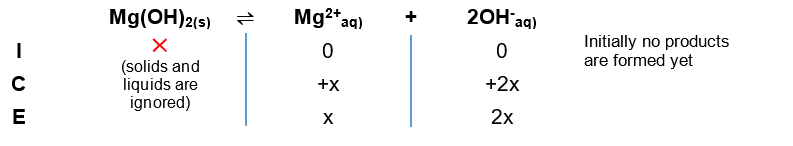# Problem: Calculate the solubility of Mg(OH)2(s) in g/L in an aqueous solution buffered at pH = 8.60. The Ksp of Mg(OH)2 is 5.61 x 10-12.

###### FREE Expert Solution

STEP 1: Recall that ionic compounds such as Mg(OH)form ions when dissociating in water.

Magnesium is in Group 2A so it’s charge is +2; The hydroxide ion, OHhas a charge of –1.

Mg(OH)2(s)  Mg2+(aq) + 2OH(aq)

**OH- has a subscript of 2 in Mg(OH)2 so it forms 2 OH- ions when it dissociates

STEP 2: Make an ICE Chart**We lose reactants (-x) and gain products (+x)

The equilibrium expression for the solubility product constant, Ksp is:

$\overline{){{K}}_{sp}{=}\frac{\left[products\right]}{\overline{)\left[reac\mathrm{tan}ts\right]}}{=}\left[M{g}^{2+}\right]{\left[O{H}^{-}\right]}^{{2}}}$

**Solids are ignored in Ksp expression

**Concentrations must be raised by the stoichiometric coefficient

90% (467 ratings)###### Problem Details

Calculate the solubility of Mg(OH)2(s) in g/L in an aqueous solution buffered at pH = 8.60. The Ksp of Mg(OH)2 is 5.61 x 10-12.